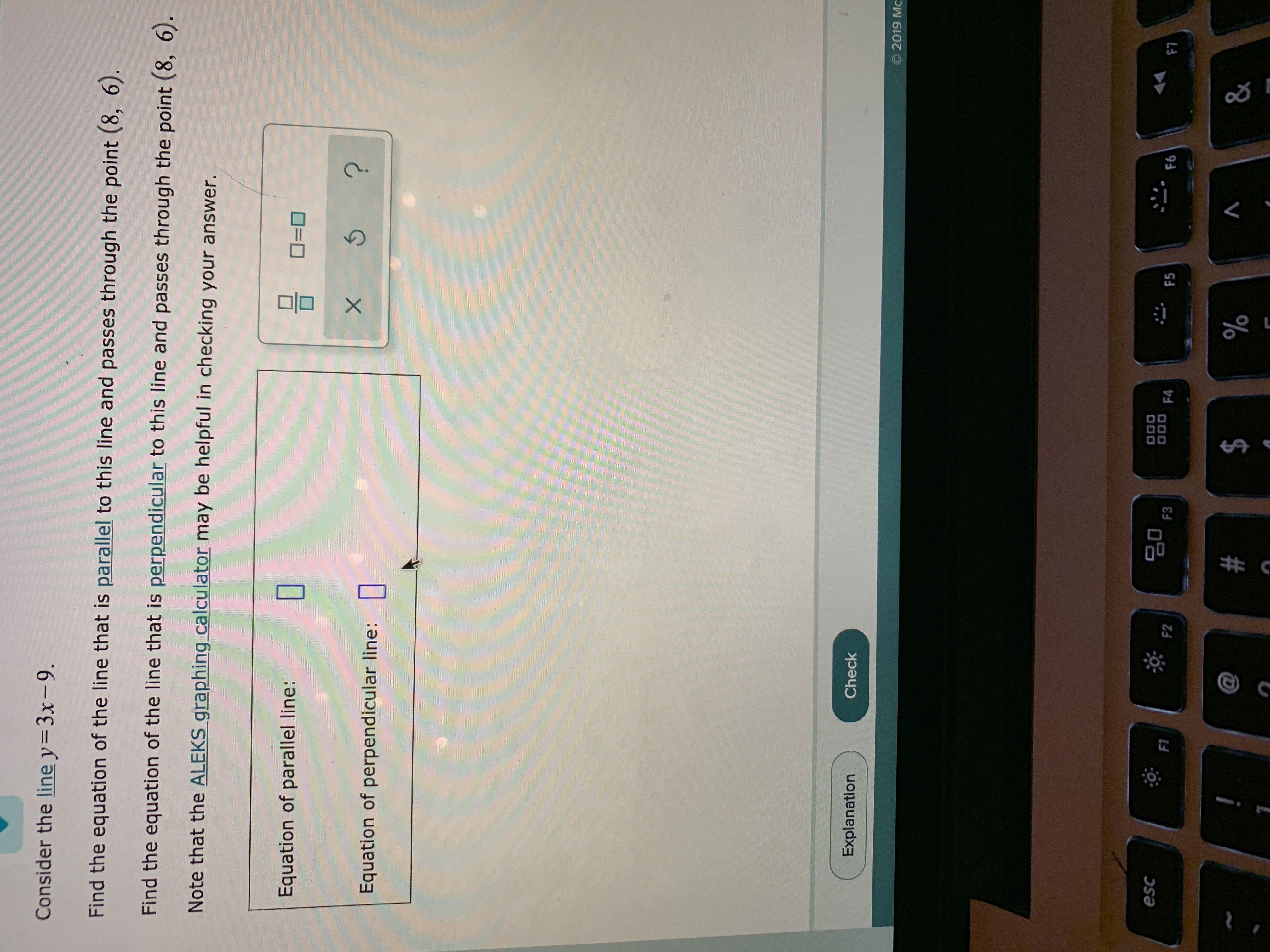# Consider the line y= 3x- 9.Find the equation of the line that is parallel to this line and passes through the point (8, 6).Find the equation of the line that is perpendicular to this line and passes through the point (8, 6).Note that the ALEKS graphing calculator may be helpful in checking your answer.Equation of parallel line:X 5Equation of perpendicular line:CheckExplanationO 2019 Mc000DO0 FAescF7F6F5F3F1F2&\$#7

Question
10 viewshelp_outlineImage TranscriptioncloseConsider the line y= 3x- 9. Find the equation of the line that is parallel to this line and passes through the point (8, 6). Find the equation of the line that is perpendicular to this line and passes through the point (8, 6). Note that the ALEKS graphing calculator may be helpful in checking your answer. Equation of parallel line: X 5 Equation of perpendicular line: Check Explanation O 2019 Mc 000 DO0 FA esc F7 F6 F5 F3 F1 F2 & \$ # 7 fullscreen
check_circle

Step 1

Calculation:

The given line is observed to be in the form of y = mx + b, where m is 3.

Compute the equation of the line parallel to y = 3x – 9 as follows.

Since the lines are parallel, their slopes will be equal.

Substitute (x1, y1) = (8, 6) and m = 3 in (y y1) = m(x x1)

(y –...

### Want to see the full answer?

See Solution

#### Want to see this answer and more?

Solutions are written by subject experts who are available 24/7. Questions are typically answered within 1 hour.*

See Solution
*Response times may vary by subject and question.
Tagged in

### Other